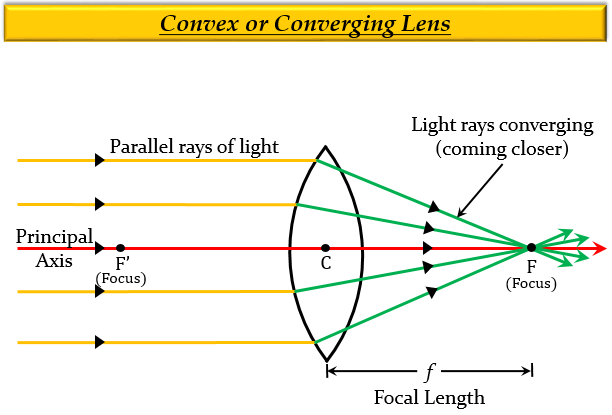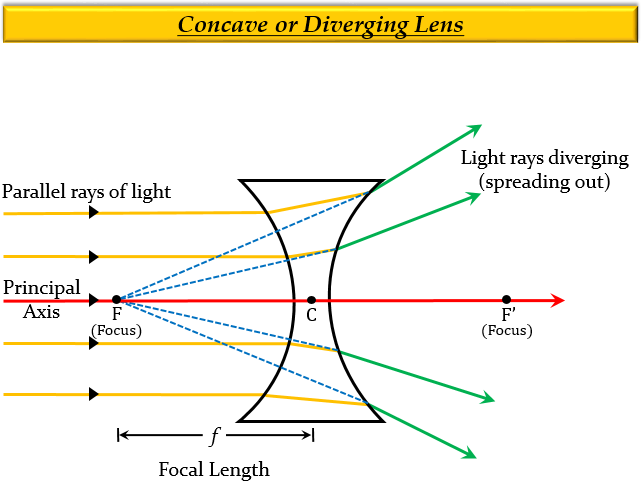# Which type of lens is : (a) a converging lens, and which is (b) a perging lens? Explain your answer with diagrams.

(a) Converging Lens or Convex Lens $-$ It is a lens that converges a parallel beam of light rays passing through it at its focus.

The ray diagram given below shows the parallel light rays converging at the focus of a convex lens.Explanation

Converging Lens or Convex Lens $-$ It is a lens that possesses at least one surface that curves outwards. In other words, it is thick across the middle and thin at the upper and lower edges, because of which the light that enters the lens, gets focused, or converged. Due to this effect, it is also known as a positive lens or a converging lens.

The image formed by a convex lens is both real and virtual depending on the position of the object, which means both diminished and magnified images can be produced.

(b) Diverging Lens or Concave Lens $-$ It is a lens which diverges a parallel beam of light passing through it.

The ray diagram given below shows the parallel light rays getting diverged when passed through a concave lens.Explanation

Diverging Lens or Concave Lens $-$ It is a lens that possesses at least one surface that curves inwards in the middle. In other words, it is thin across the middle and thick at the upper and lower edges, because of which the light that enters the lens, gets spread out, or diverges, which results in forming a smaller image. Due to this effect, it is also called a negative lens or a diverging lens.

The image formed by a concave lens is virtual & erect, which means it will appear to be farther away than it actually is, and therefore smaller than the object itself.

Updated on: 10-Oct-2022

35 Views